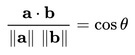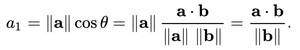### IntroductionIf a and b are two vectors, the vector projection of a on b is the vector a1 with the same direction as b and with the length:When θ is not known, we can compute cos⁡θ using the following property of Dot Product a∙b:Thus, the length of a1 can be also computed as follows:Since a1 is in the same direction as b,Whereis the Unit Vector with the same direction as b:### References & Resources

• Wikipedia - http://en.wikipedia.org/wiki/Vector_projection